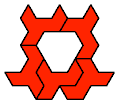# Polyform Tiling

The slabs made a most intricate and fascinating design, but a thoroughly unobtrusive one, unless one paid deliberate attention to it.
—Carlos Castaneda, The Second Ring of Power
The tiling problem is to join copies of one or more polyforms to make a given polyform.
• Plane Tiling
• Solid Tiling
• ## Plane Tiling

• Polyominoes and Polykings
• Polyiamonds
• Polyaboloes/Polytans and Polyfetts/Polifetti
• Polydrafters
• Polydoms
• Various
• ### Polyominoes and Polykings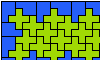Two-Pentomino Balanced Rectangles. Tile a rectangle with two pentominoes in equal quantities.Two-Pentomino Holey Balanced Rectangles. Tile a rectangle with two pentominoes in equal quantities, allowing one-cell holes.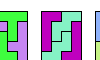Scaled Two-Pentomino Rectangles. Tile a rectangle with two pentominoes at various sizes.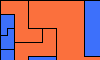Scaled Two-Pentomino Balanced Rectangles. Tile a rectangle with various sizes of two pentominoes in equal areas.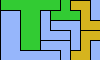Scaled Three-Pentomino Rectangles. Tile a rectangle with three pentominoes at various sizes.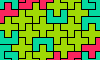Three-Pentomino Balanced Rectangles. Tile a rectangle with three pentominoes in equal quantities.Scaled Three-Pentomino Balanced Rectangles. Tile a rectangle with various sizes of three pentominoes in equal areas.L Shapes From Two Pentominoes. Form an L-shaped (hexagonal) polyomino with copies of two pentominoes, using at least one of each.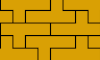Prime Rectangle Tilings for the Y Pentomino. Irreducible rectangles formed of Y pentominoes.Tiling a Beveled Rectangle with Polyominoes.Yin-Yang Dominoes. Arrange 10 of the 12 pentominoes to cover a bi-colored domino.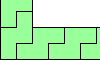Tiling Strips with Polyominoes. Tiling straight, bent, branched, and crossed infinite strips with polyominoes of orders 1 through 6.Uniform Polyomino Stacks. Join copies of a polyomino to make a figure with uniform row width.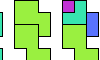Perfect Polyominoes. Polyominoes that can be formed by joining all the smaller polyominoes that can tile them.Polyomino Bireptiles. Join two copies of a polyomino, then dissect the result into equal smaller copies of it.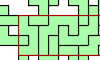Covering a Rectangle with Copies of a Polyomino. Find the largest rectangles that copies of a polyomino can cover without overlapping.Prime Rectangles for Tetrakings.. For each tetraking, find the irreducible rectangles that it can tile.

### Polyiamonds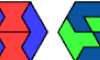Two-Hexiamond Balanced Hexagons. Tile a regular hexagon with two hexiamonds in equal quantities.Polyiamond Hexagon Tiling. Tile a straight or ragged hexagon with various polyiamonds.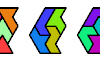Hexiamond Triplets. Arrange the 12 hexiamonds to form three congruent polyiamonds.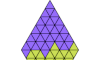Yin-Yang Diamonds. Arrange the 12 hexiamonds to cover a bi-colored diamond.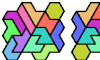Tiling a Polyhex with the 12 Hexiamonds. Arrange the 12 hexiamonds to form a polyhex.Similar Hexiamond Figures, 2–2–8. With the 12 hexiamonds, make three similar figures, one at double scale.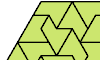Minimal Convex Polyiamond Tilings. With copies of a given polyiamond make the smallest convex polyiamond.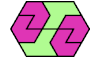Convex Polygons from Pairs of Polyiamonds. With copies of two given polyiamonds make the smallest convex polyiamond.Convex Polygons from Three Hexiamonds. With copies of three given hexiamonds make the smallest convex polyiamond.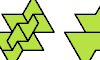Polyiamond Bireptiles. Join two copies of a polyiamond, then dissect the result into equal smaller copies of it.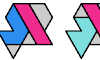Containing Pairs of Hexiamonds. Find the smallest polyiamonds that can contain every pair of distinct hexiamonds.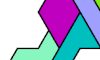Tiling a Shape with Ternary Symmetry with the Heptiamonds and the Tetrahexes. Tile a shape with 3-fold symmetry with all 24 heptiamonds, then with all 7 tetrahexes.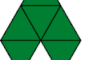The Lobster and the Snake. Four puzzles about the Lobster and Snake hexiamonds.

### Polyaboloes/Polytans and Polyfetts/PolifettiPolyabolo Irreptiles. Join variously sized copies of a polyabolo to make a replica of itself.Similar Polyaboloes Tiling a Triangle. Join variously sized copies of a polyabolo to make a triangle.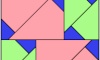Similar Polyaboloes Tiling a Square. Join variously sized copies of a polyabolo to make a square.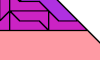Similar Polyaboloes Tiling a Right Trapezoidal Triabolo. Join variously sized copies of a polyabolo to make a right trapezoidal triabolo.Similar Polyaboloes Tiling an Octagon. Join variously sized copies of a polyabolo to make an octagon.Similar Polyaboloes Tiling a Home Plate Hexabolo. Join variously sized copies of a polyabolo to make a home plate.Similar Polyaboloes Tiling a Crown Heptabolo. Join variously sized copies of a polyabolo to make a crown heptabolo.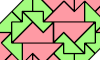Similar Polyaboloes Forming a Convex Shape. Join variously sized copies of a polyabolo to make a convex shape.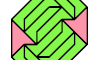Convex Polygons from Pairs of Polytans. With copies of two given polytans make the smallest convex polytan.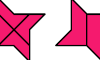Polytan Bireptiles. Join two copies of a polytan, then dissect the result into equal smaller copies of it.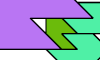Scaled Polytan Tetrads. Join four similar polytans so that each borders the other three.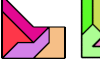Similar Pentatan Figures 2–2√2–3√2. Arrange the 30 pentatans to make three copies of the same pentatan at scales 2, 2√2, and 3√2.Tiling a Polytan With Unequal Monotans. Dissect an arbitrary polytan into isosceles right triangles, all of different sizes.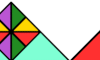Polyfett Irreptiles. Tile a polyfett with smaller copies of itself, not necessarily equal.Similar Polyfetts Tiling a Triangle. Tile a triangle with variously sized copies of a polyfett.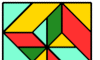Similar Polyfetts Tiling a Square. Tile a square with variously sized copies of a polyfett.

### Polydrafters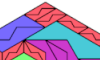Polydrafter Irreptiling. Tile a polydrafter with smaller copies of itself, not necessarily equal.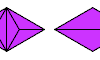Polydrafter Bireptiles. Join two copies of a polydrafter, then dissect the result into equal smaller copies of it.The Didrafter Fish. Form a compact shape with the 13 proper and extended didrafters.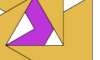Scaled Polydrafter Tetrads. Join four similar polydrafters so that each borders the other three.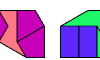Convex Figures with Didrafter Pairs. Make a convex polydrafter with copies of two didrafters.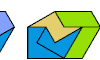Convex Figures with Didrafter Triplets. Make a convex polydrafter with copies of three didrafters.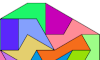Convex Shapes from the 13 Didrafters. Make a convex polydrafter with the 13 didrafters.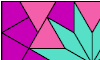Rectangles Tiled with Three Didrafters. Make a rectangle with copies of three didrafters.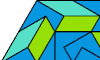Regular Hexagons Tiled with Three Didrafters. Make a regular hexagon with copies of three didrafters.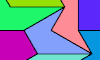Making a Rectangle from Different Didrafters. Make a rectangle out of up to eight distinct didrafters.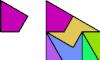Didrafters at Scales 1 and 5. Arrange a double set of the 13 didrafters to form copies of a didrafter at scales 1 and 5.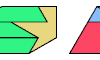Convex Figures with Tridrafter Pairs. Make a convex shape with copies of two tridrafters.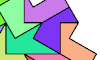Galaxies from the 14 Tridrafters. Join the 14 proper tridrafters to make a shape with 6-rotary symmetry.Stelo Twins and Triplets. Use Jacques Ferroul's Stelo pieces to make multiple copies of the same shape.

### Polydoms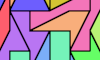Kiteless Didoms. Form shapes with the set of 12 didoms, omitting the kite didom.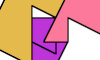Scaled Polydom Tetrads. Join four similar polydoms so that each borders the other three.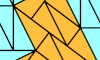A Counterexample To Livio Zucca's Island Conjecture. Make a polydom island in the shape of a polyomino that cannot be tiled with dominoes.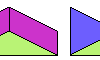Convex Figures with Didom Pairs. Make a convex polydom with copies of two didoms.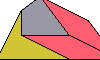Convex Figures with Didom Triplets. Make a convex polydom with copies of three didoms.Convex Shapes from the 13 Didoms. Make a convex polydom with the 13 didoms, including the Kite Didom.Tiling the Owen Dodecagon With Three Didoms. Make an almost regular dodecagon with copies of the 13 didoms.

### VariousContiguous Partridge Tilings. Use 1 shape at scale 1, 2 at scale 2, and so on up to n at scale n, to form a scaled copy of the shape in which equal tiles are contiguous.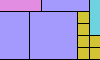Contiguous Reverse Partridge Tilings. Use 1 shape at scale n, 2 at scale n−1, and so on up to n at scale 1, to form a scaled copy of the shape in which equal tiles are contiguous.Lovebirds Tilings. Use 2 copies of a shape at scale 1, 4 at scale 2, and so on up to 2n at scale n, to form two scaled copies of the shape.

## Solid TilingPentacubes in a Box. Join copies of a pentacube to make a rectangular prism.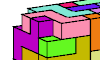Pentacubes in a Box Without Corners. Join copies of a pentacube to make a rectangular prism with its corner cells removed.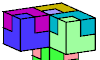Polycube Reptiles. Join copies of a polycube to make a larger copy of itself.Polycube Bireptiles. Join two copies of a polycube, then dissect the result into equal smaller copies of it.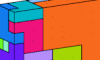Proper Minimal Polycube Irreptiles. Join variously sized copies of a polycube to make a larger copy of itself, using fewer copies than would be needed if they were all the same size.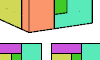33 + 43 + 53 = 63. Dissect a cube of side 6 to make cubes of sides 3, 4, and 5.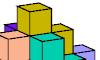Tiling a Solid Diamond Polycube With Right Tricubes. Dissect an octahedron-shaped polycube into L-shaped tricubes.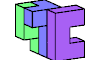Symmetric Pentacube Triples. Join three different pentacubes to form a symmetric polycube.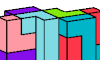Polycube Prisms. Join copies of a polycube to make a prism.Pentacube Pair Pyramids. Join copies of two polycubes to make a pyramid.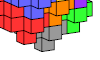Filling Space with the Pansymmetric Heptacube. Use the 3D analogue of an X pentomino to fill space.Tiling a Scaled Pentacube with a Pentacube. Use a pentacube to tile various pentacubes scaled up by 2 or 3.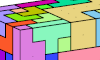Inflated Pentacubes. Make a box with the 29 pentacubes, enlarging one of them by a scale factor of 2.

Back to Polyform Curiosities
Col. George Sicherman [ HOME | MAIL ]